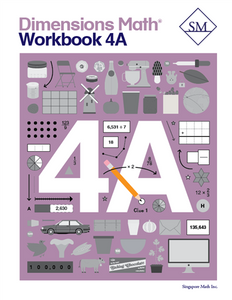# Dimensions Math Workbook 4A

Vendor
Singapore Math Inc.
Regular price
\$13.70
Sale price
\$13.70
Quantity must be 1 or more

The Dimensions Math® PK-5 series is a new curriculum designed by Singapore Math Inc. to better serve U.S. teachers and students. Aspects of Singapore math curriculum have been updated for clarity and relevance, while preserving the solid foundation that makes it unique. The series follows the principles outlined in the Singapore Mathematics Framework and uses the Concrete > Pictorial > Abstract approach. The curriculum also incorporates Singapore mental math strategies and model drawing.

In the Dimensions Math program, concepts are introduced and explained in a new format with vibrant imagery. The implementation of this curriculum suits today’s needs, while the progression and scope that define Singapore math remain intact.

The Dimensions Math Workbook 4A corresponds to the 4A textbook (required and sold-separately). Exercises start with simpler problems and progresses to more challenging exercises. A thoughtful progression pattern followed throughout the series covers the fundamental concepts, offers practice exercises with pictorial representations, and challenges students with abstract exercises. This Grade 4 level workbook covers numbers to one million, addition and subtraction, multiples and factors, multiplication, division, fractions, adding and subtracting fractions, multiplying a fraction and a whole number, and line graphs and line plots. Exercises align with the text, and include "basics," "practice," "check," and "challenge" questions.